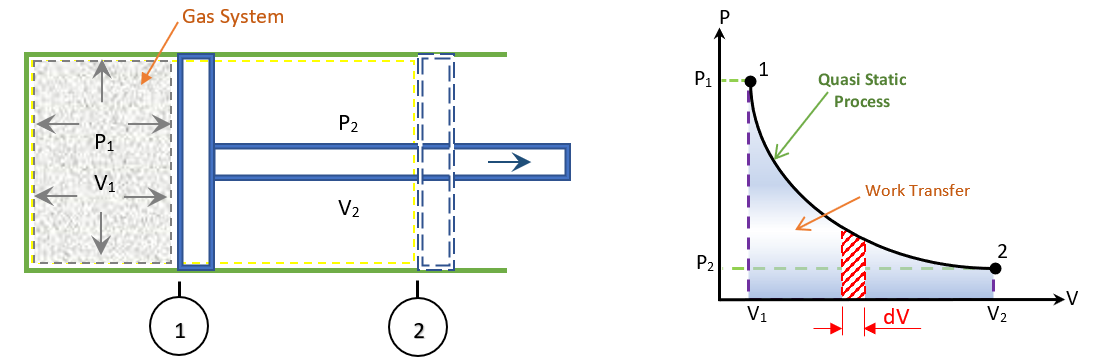### Displacement WorkFigure: Displacement Work or pdV Work

• Consider a system as shown in figure initially at position-1 having pressure ‘p1’ and volume ‘V1’. The gas system is in thermodynamic equilibrium described by the coordinates ‘p1 and V1’. The piston is the only boundary which moves due to gas pressure. Let the piston now move to a new final position-2 which is also a thermodynamic equilibrium state specified by pressure ‘p2’ and volume ‘V2’.

• Picture: Displacement Work

• When the piston moves a infinitesimal distance ‘dL’ and if ‘A’ be the area of the piston, the force acting on the piston is-
F = p x A..........1

• And the infinitesimal amount of work done by the gas on piston-
ᵭW = F x dL

• By Definition of Pressure; we have p = F / A
ᵭW = p x A x dL
ᵭW = pdV..........2
Where: dv = A x dL – Infinitesimal displacement volume.

• When the piston move from position-1 to position-2 with the volume changing from V1 to V2, the amount of work done ‘W’ by the system will be-
..........3

• The magnitude of work done is given by the area under the path 1-2, as shown in figure. Since ‘p’ is at all times a thermodynamic coordinate all the states passed through by the system as the volume change from V1 to V2 must be equilibrium states and the path 1-2 must be quasi-static. The piston moves infinitely slowly so that every state passes through an equilibrium state. The ∫pdV can be performed only on a quasi-static path.

Limitations of using ∫Pdv
The following conditions must be satisfied for ∫pdV to represent the work done. They are-

• The system should be a closed one

• The process must be quasi-static

• The boundary of the system should move in order that work is done either by the system or on the system